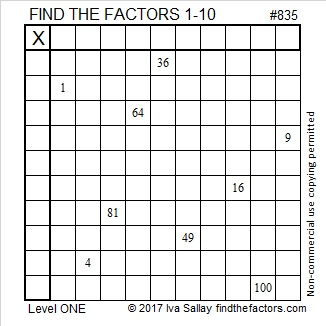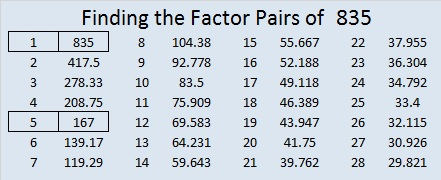# 835 and Level 1

835 is the hypotenuse of a Pythagorean triple, 501-668-835, which is 167 times (3-4-5).

835 can be written as the difference of two squares two different ways:

• 418² – 417² = 835
• 86² – 81² = 835

835 can be written as the sum of consecutive numbers three different ways.

• 417 + 418 = 835; that’s two consecutive numbers.
• 165 + 166 + 167 + 168 + 169 = 835; that’s five consecutive numbers.
• 79 + 80 + 81 + 82 + 83 + 84 + 85 + 86 + 87 + 88 = 835; that’s ten consecutive numbers.Print the puzzles or type the solution on this excel file: 10-factors-835-842

• 835 is a composite number.
• Prime factorization: 835 = 5 × 167
• The exponents in the prime factorization are 1 and 1. Adding one to each and multiplying we get (1 + 1)(1 + 1) = 2 × 2 = 4. Therefore 835 has exactly 4 factors.
• Factors of 835: 1, 5, 167, 835
• Factor pairs: 835 = 1 × 835 or 5 × 167
• 835 has no square factors that allow its square root to be simplified. √835 ≈ 28.89636655This site uses Akismet to reduce spam. Learn how your comment data is processed.